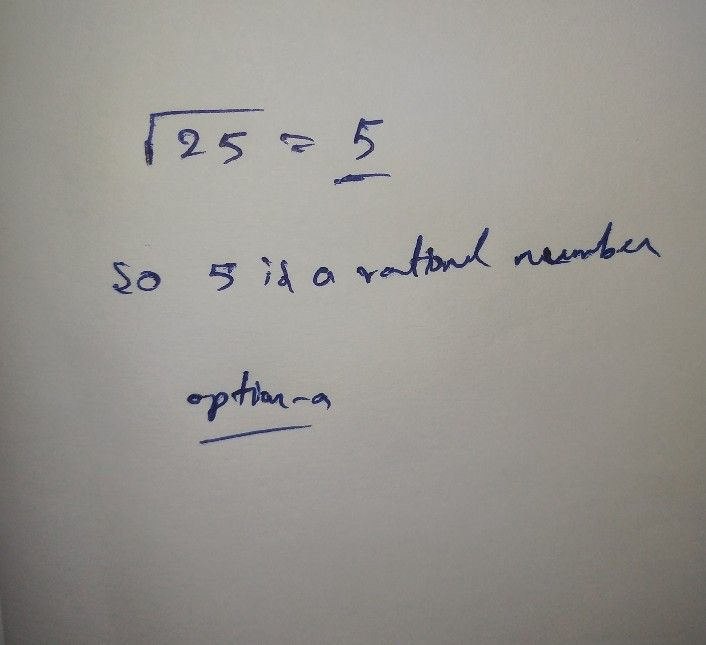Symbol
Problem$2\right)$ Which of the following is rational no. * $○$ $s9rt\left(25\right)$ $○$ $s9rt\left(101\right)$ $○$ O $s9r+\left(152\right)$
7th-9th grade
Algebra
SolutionQanda teacher - Aishwarya